Next: 11.2.2.2 Using Dual-Paraboloid Maps Up: 11.2.2 Dual-Paraboloid Environment Mapping Previous: 11.2.2 Dual-Paraboloid Environment Mapping   Contents

#### 11.2.2.1 The Mathematics of Dual-Paraboloid Maps

The principle that underlies paraboloid maps is the same principle that underlies a parabolic lens or satellite dish. The geometry of a paraboloid can focus rays. The paraboloid used for dual-paraboloid mapping is: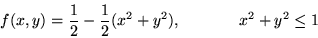Figure 74 shows how two paraboloids can focus the entire environment surrounding a point into two images.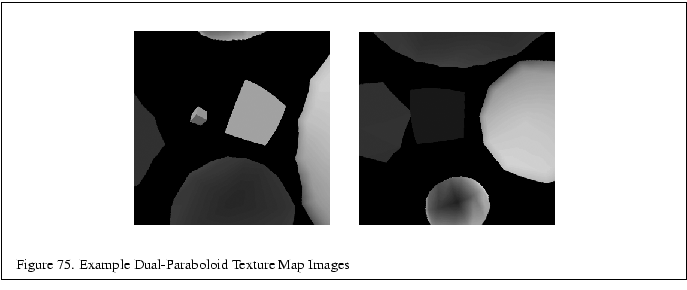Unlike the sphere mapping approach that the encodes the entire environment in a single texture, the dual-paraboloid mapping scheme requires two textures to store the environment, one texture for the front'' environment and another texture for the back'' environment. Importantly, the sense of front'' and back'' is completely independent of the viewer orientation. Figure 75 shows an example of two paraboloid maps. Because two textures are required, the technique must be performed in two rendering passes though this can be reduced to a single rendering pass if multitexturing is supported.

Because the math for the paraboloid is all linear (unlike the spherical basis of the sphere map), Heidrich and Seidel observe that OpenGL with its texture matrix can map a eye-coordinate reflection vector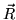into a 2D texture coordinate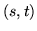within a dual-paraboloid map. Construct the necessary texture matrix as follows: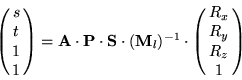where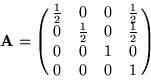is a matrix that scales an biases a 2D coordinate in the range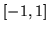to the texture image range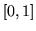. And where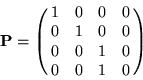is a projective transform that divides by the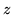coordinate. This serves to flatten a 3D vector into 2D. And where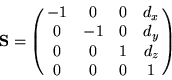is a matrix that subtracts the supplied 3D vector from an orientation vector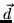that supplies a view direction. We will makeeither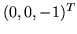ordepending on whether we are mapping the front or back paraboloid map respectively. Finally, the matrix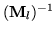is the inverse of the linear part of the current (affine) modelview matrix. The matrixtransforms a 3D eye-space reflection vector into an object-space version of the vector.Next: 11.2.2.2 Using Dual-Paraboloid Maps Up: 11.2.2 Dual-Paraboloid Environment Mapping Previous: 11.2.2 Dual-Paraboloid Environment Mapping   Contents
2001-01-10